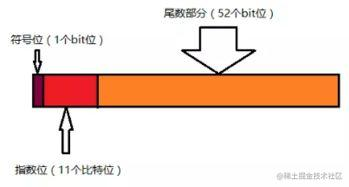# 解决JS浮点数运算结果不精确的Bug

最近在做项目的时候，涉及到产品价格的计算，经常会出现JS浮点数精度问题，这个问题，对于财务管理系统的开发者来说，是个非常严重的问题（涉及到钱相关的问题都是严重的问题），这里把相关的原因和问题的解决方案整理一下，也希望给各位提供一些参考。

## 一. 常见例子

``````   // 加法
0.1 + 0.2 = 0.30000000000000004
0.1 + 0.7 = 0.7999999999999999
0.2 + 0.4 = 0.6000000000000001

// 减法
0.3 - 0.2 = 0.09999999999999998
1.5 - 1.2 = 0.30000000000000004

// 乘法
0.8 * 3 = 2.4000000000000004
19.9 * 100 = 1989.9999999999998

// 除法
0.3 / 0.1 = 2.9999999999999996
0.69 / 10 = 0.06899999999999999

// 比较
0.1 + 0.2 === 0.3 // false
(0.3 - 0.2) === (0.2 - 0.1) // false复制代码``````## 二. 导致原因

JavaScript 内部只有一种数字类型Number，也就是说，JavaScript 语言的底层根本没有整数，所有数字都是以IEEE-754标准格式64位浮点数形式储存，`1``1.0`是相同的。因为有些小数以二进制表示位数是无穷的。JavaScript会把超出53位之后的二进制舍弃，所以涉及小数的比较和运算要特别小心。

## 三. IEEE二进制浮点数算术标准（IEEE 754）

IEEE二进制浮点数算术标准IEEE 754）是20世纪80年代以来最广泛使用的浮点数运算标准，为许多CPU与浮点运算器所采用。这个标准定义了表示浮点数的格式（包括负零-0）与反常值（denormal number）），一些特殊数值（无穷（Inf）与非数值（NaN）），以及这些数值的“浮点数运算符”；它也指明了四种数值舍入规则和五种例外状况（包括例外发生的时机与处理方式）。

## 四. 浮点数的存储

JS的浮点数实现也是遵循IEEE 754标准，采用双精度存储（double precision），使用64位固定长度来表示，其中1位用来表示符号位，11位用来表示指数，52位表示尾数。如下图：• 符号位(sign)：第1位是正负数符号位，0代表正数，1代表负数
• 指数位(Exponent)：中间11位存储指数，用来表示次方数
• 尾数位(mantissa)：最后的52位是尾数，超出部分自动进一舍零

## 五. 浮点数的计算步骤（0.1+0.2）

【1】首先，十进制的0.1和0.2会转换成二进制的，但是由于浮点数用二进制表示是无穷的

`````` 0.1——>0.0001 1001 1001 1001 ...(1001循环)
0.2——>0.0011 0011 0011 0011 ...(0011循环)复制代码``````【2】IEEE754标准的64位双精度浮点数的小数部分最多支持53位二进制，多余的二进制数字被截断，所以两者相加之后的二进制之和是

`` 0.0100110011001100110011001100110011001100110011001101复制代码``【3】将截断之后的二进制数字再转换为十进制，就成了0.30000000000000004，所以在计算时产生了误差

## 六. 解决办法

【1】引用类库

【2】思路一：在知道小数位个数的前提下，可以考虑通过将浮点数放大倍数到整型(最后再除以相应倍数)，再进行运算操作，这样就能得到正确的结果了

``````   0.1 + 0.2 ——> (0.1 * 10 + 0.2 * 10) / 10 // 0.3
0.8 * 3 ——> ( 0.8 * 100 * 3) / 100         //2.4复制代码``````【3】自定义一个转换和处理函数

``````    // f代表需要计算的表达式，digit代表小数位数
Math.formatFloat = function (f, digit) {
// Math.pow(指数，幂指数)
var m = Math.pow(10, digit);
// Math.round（） 四舍五入
return Math.round(f * m, 10) / m;
}
console.log(Math.formatFloat(0.3 * 8, 1));  // 2.4
console.log(Math.formatFloat(0.35 * 8, 2));  // 2.8复制代码``````【4】加法函数

``````    /**
** 加法函数，用来得到精确的加法结果
** 说明：javascript的加法结果会有误差，在两个浮点数相加的时候会比较明显。这个函数返回较为精确的加法结果。
** 返回值：arg1加上arg2的精确结果
**/
var r1, r2, m, c;
try {
r1 = arg1.toString().split(".").length;
} catch (e) {
r1 = 0;
}
try {
r2 = arg2.toString().split(".").length;
} catch (e) {
r2 = 0;
}
c = Math.abs(r1 - r2);
m = Math.pow(10, Math.max(r1, r2));
if (c > 0) {
var cm = Math.pow(10, c);
if (r1 > r2) {
arg1 = Number(arg1.toString().replace(".", ""));
arg2 = Number(arg2.toString().replace(".", "")) * cm;
} else {
arg1 = Number(arg1.toString().replace(".", "")) * cm;
arg2 = Number(arg2.toString().replace(".", ""));
}
} else {
arg1 = Number(arg1.toString().replace(".", ""));
arg2 = Number(arg2.toString().replace(".", ""));
}
return (arg1 + arg2) / m;
}

};复制代码``````【5】减法函数

``````    /**
** 减法函数，用来得到精确的减法结果
** 说明：javascript的减法结果会有误差，在两个浮点数相减的时候会比较明显。这个函数返回较为精确的减法结果。
** 调用：accSub(arg1,arg2)
** 返回值：arg1加上arg2的精确结果
**/
function accSub(arg1, arg2) {
var r1, r2, m, n;
try {
r1 = arg1.toString().split(".").length;
} catch (e) {
r1 = 0;
}
try {
r2 = arg2.toString().split(".").length;
} catch (e) {
r2 = 0;
}
m = Math.pow(10, Math.max(r1, r2)); //last modify by deeka //动态控制精度长度
n = (r1 >= r2) ? r1 : r2;
return ((arg1 * m - arg2 * m) / m).toFixed(n);
}

// 给Number类型增加一个mul方法，调用起来更加方便。
Number.prototype.sub = function (arg) {
return accMul(arg, this);
};复制代码``````【6】乘法函数

``````    /**
** 乘法函数，用来得到精确的乘法结果
** 说明：javascript的乘法结果会有误差，在两个浮点数相乘的时候会比较明显。这个函数返回较为精确的乘法结果。
** 调用：accMul(arg1,arg2)
** 返回值：arg1乘以 arg2的精确结果
**/
function accMul(arg1, arg2) {
var m = 0,
s1 = arg1.toString(),
s2 = arg2.toString();
try {
m += s1.split(".").length;
} catch (e) {}
try {
m += s2.split(".").length;
} catch (e) {}
return Number(s1.replace(".", "")) * Number(s2.replace(".", "")) / Math.pow(10, m);
}

// 给Number类型增加一个mul方法，调用起来更加方便。
Number.prototype.mul = function (arg) {
return accMul(arg, this);
};复制代码``````【7】除法函数

``````    /**
** 除法函数，用来得到精确的除法结果
** 说明：javascript的除法结果会有误差，在两个浮点数相除的时候会比较明显。这个函数返回较为精确的除法结果。
** 调用：accDiv(arg1,arg2)
** 返回值：arg1除以arg2的精确结果
**/
function accDiv(arg1, arg2) {
var t1 = 0,
t2 = 0,
r1, r2;
try {
t1 = arg1.toString().split(".").length;
} catch (e) {}
try {
t2 = arg2.toString().split(".").length;
} catch (e) {}
with(Math) {
r1 = Number(arg1.toString().replace(".", ""));
r2 = Number(arg2.toString().replace(".", ""));
return (r1 / r2) * pow(10, t2 - t1);
}
}

//给Number类型增加一个div方法，调用起来更加方便。
Number.prototype.div = function (arg) {
return accDiv(this, arg);
};复制代码``````